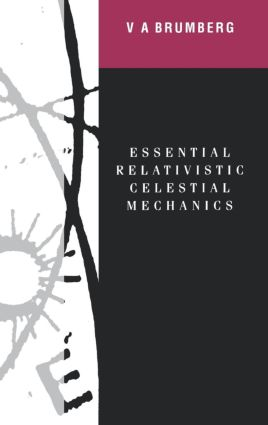Essential Relativistic Celestial Mechanics

1st Edition

CRC Press

274 pages | 121 B/W Illus.

Purchasing Options:\$ = USD
Hardback: 9780750300629
pub: 1991-01-01
SAVE ~\$53.00
\$265.00
\$212.00
x
eBook (VitalSource) : 9780203756591
pub: 2017-11-22
from \$28.98

FREE Standard Shipping!

Description

Essential Relativistic Celestial Mechanics presents a systematic exposition of the essential questions of relativistic celestial mechanics and their relation to relativistic astrometry. The book focuses on the comparison of calculated and measurable quantities that is of paramount importance in using general relativity as a necessary framework in the discussion of high-precision observations and for the construction of accurate dynamical ephemerides. It discusses the results of the general relativistic theory of motion of celestial bodies and describes the relativistic theory of astronomical reference frames, time scales, and the reduction of observations.

MATHEMATICAL TOOLS

Elements of Newtonian celestial mechanics

Elements of Riemannian geometry and tensor analysis

Elements of special theory of relativity

GRT FIELD EQUATIONS

Basic principles of GRT

Weak gravitational field

Problem of measurable quantities in GRT

ONE-BODY PROBLEM

Schwarzschild problem

Light propagation in the Schwarzschild problem

Field of rotating spheroid

APPROXIMATE SOLUTIONS OF THE FIELD EQUATIONS AND APPROXIMATE EQUATIONS OF MOTION

N-body problem

Geocentric reference frame

Equations in variations for the spherically symmetrical metric spaces

Gravitational radiation and motion in binary system

EQUATIONS OF MOTION OF SOLAR SYSTEM BODIES

Equations of motion of Earth's artificial satellites

Motion of the major planets

Motion of the Moon

RELATIVISTIC REDUCTION OF ASTROMETRIC MEASUREMENTS

General principles of reduction

Relativistic theory of astronomical reference systems

Relativistic reduction of astrometric observations

Relativistic reduction of radio observations

RELATIVISTIC EFFECTS IN GEODYNAMICS

Timescales on Earth

Clocks and satellites in the circumterrestrial space

Postcript

References

Index